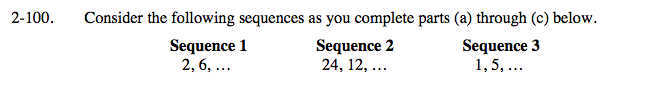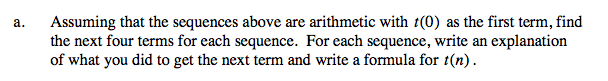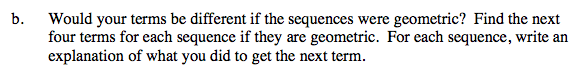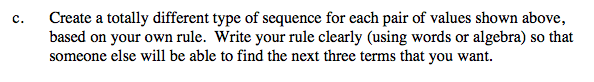### Home > CAAC > Chapter 14 > Lesson 14.2.1.7 > Problem2-100

2-100.
1. Consider the following sequences as you complete parts (a) through (c) below. Homework Help ✎

 Sequence 1 2, 6, … Sequence 2 24, 12, … Sequence 3 1, 5,…
1. Assuming that the sequences above are arithmetic with t(0) as the first term, find the next four terms for each sequence. For each sequence, write an explanation of what you did to get the next term and write a formula for t(n).

2. Would your terms be different if the sequences were geometric? Find the next four terms for each sequence if they are geometric. For each sequence, write an explanation of what you did to get the next term.

3. Create a totally different type of sequence for each pair of values shown above, based on your own rule. Write your rule clearly (using words or algebra) so that someone else will be able to find the next three terms that you want.Sequence 1:

6 − 2 = 4

Add 4 to each term to get the next.

The next 4 terms are 10, 14, 18, 22.

t(n) = 4n + 2Sequence 1:

$\frac{6}{2}=3$

Multiply each term by 3 to get the next.

The next 4 terms are 18, 54, 162, 486.